# Using RSum for the HP 38G

The RSum program calculates the left-hand, right-hand, trapezoid and midpoint Riemann sums which are commonly used to estimate the value of a single definite integral of a reasonably well-behaved real-valued function.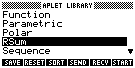1. To start Rsum, press the LIB key. The resulting display should look similar to theone on the right. Highlight Rsum, then press the START key.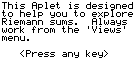2. When you start RSum, after a brief copyright message, you will see the screen on theright. Read and follow the directions.3. When the screen on the right appears, enter the integrand (the function to beintegrated) into the 'F1(X)' field. PLEASE NOTE: The integrand MUST be F1(X);F2...F0 are currently not useable. Futhermore, you must make certain that F1(X) is 'checked',so that it is activated for graphing.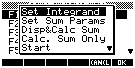4. Now, to begin using the Aplet, press the VIEWS key. When you do so, you will see themenu on the right displayed. RSum's FUNCTIONALITY IS ACCESSIBLE ONLY FROM THIS MENU. 'SetIntegrand' allows you to choose another integrand - for now, let's keep the one that youentered in step 3 (above). Instead, choose 'Set Sum Params' by highlighting it, thenpressing the OK key.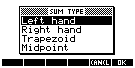You will then see the prompt screen on the right. Highlight 'Left Hand' (for the lefthand sum), then press the OK key again. The final screen asks for the limits ofintegration (from 'H-MIN', on the left, to 'H-MAX', on the right) and the number of strips(the sub-areas, '# of Subs') to be used. These must be entered as a list (enclosed inbraces) and in the order described. When you press OK , the graph of the portion of theintegrand within [H-MIN, H-MAX] is sketched in the display.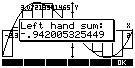5. Now, select VIEWS again. To view the approximating area strips superimposed on thegraph of the integrand, choose 'Disp&Calc Sum'. The calculated value of the Riemannsum is superimposed on this display. (Pressing any key once will remove the sum display,leaving only the sketch.) To the right is the initial result display for a left handed sumapproximation to the integral of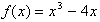on[H-MIN, H-MAX] = [-2.3, 2.0] using 13 sub-areas,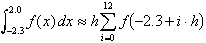for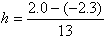.

6. Now, select VIEWS again. To calculate the Riemann sum without plotting,choose 'Calc. Sum Only'. The calculated value of the Riemann sum is displayed (much morequickly than in 5 above) in a message box. Both 'Disp&Calc Sum' and 'Calc. Sum Only'store their calculated result in the variable 'S'; so it is available in the HOME mode bytyping the variable name, 'S', followed by the ENTER key.

Both 'Disp&Calc Sum' and 'Calc. Sum Only' can be used for calculations involvingany number of subareas; however, the drawing of the large numbers of subareas istime-consuming and produces a relatively uninteresting sketch. For large numbers ofsubdivisions, 'Calc. Sum Only' returns the value of the sum much faster than does'Disp&Calc Sum'.

09/05/96(jac)